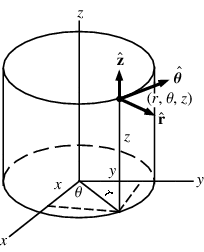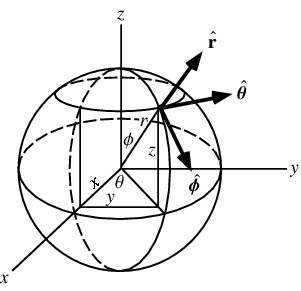# How to visualize in spherical and cylindrical coordinates

queenstudy

## Homework Statement

i just want to know how to visualize in spherical and cylindrical coordinates im really having a rough time doing that
for example why is that when we keep r constant we get a sphere and θ constant a cone why???????????

## The Attempt at a Solution

Homework Helper

The geometry follows from their definitions.

In spherical coordinates, you are just imagining you are at the origin of the coordinate system and you locate a particular position by how much you must turn to look at it and how far away it is. This is actually how you would naturally look for things IRL.

By comparison, in rectangular coords you are figuring how far you have to go in each direction of an artificial grid to reach the position.

By definition - a sphere is the set of all points in 3D that are a fixed distance from a reference point. The reference point is called the center of the sphere and the fixed distance is called the radius of the sphere.

In spherical coords, therefore, if you are at the center, the distance to any point on the surface is going to be fixed at the radius of the sphere. (If we don't put the origin of the coordinate system at the center of the sphere, then the r component will vary.)

You'll find a similar thing when you consider other shapes.
Start with the definition of a cone, and figure out how far away the surface is for someone sitting at the apex.

Everyone pretty much gets used to these things by sketching the situation.

Homework Helper
MHB

Welcome to PF, queenstudy!Here's a picture of cylindrical coordinates:If you keep r constant, you're tracing the cylinder that you're actually seeing (assuming you vary theta and z).

And here's a picture of spherical coordinates:Keeping r constant, while varying the other coordinates traces the sphere.

Keeping phi constant, while varying theta, traces the upper circle.
If we also vary r, we get a cone.

queenstudy

i understand what you people said , but the problem is that when i keep r constant for instance , if i change the angle or z like i would have to change it like 1000000000 times to get the figure
i memorized them but still having problems like how in the world would i know that when i vary r ill get a cone can a person actually imagine that???

Homework Helper
MHB

i understand what you people said , but the problem is that when i keep r constant for instance , if i change the angle or z like i would have to change it like 1000000000 times to get the figure

Yes.

i memorized them but still having problems like how in the world would i know that when i vary r ill get a cone can a person actually imagine that???

Well, I recommend you try to draw a picture.
For instance, start with the upper circle from the spherical coordinates (corresponds to varying theta while keeping r constant).
Place a dot where the origin is.
And draw a line to connect the origin to the left side of the circle (corresponds to varying r while keeping theta constant).
And another line to the right side of the circle.

Does the result look like a cone to you?

Last edited: